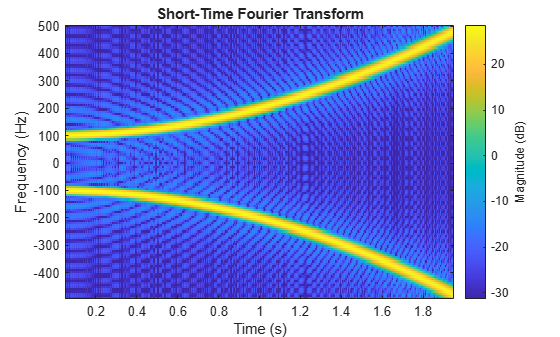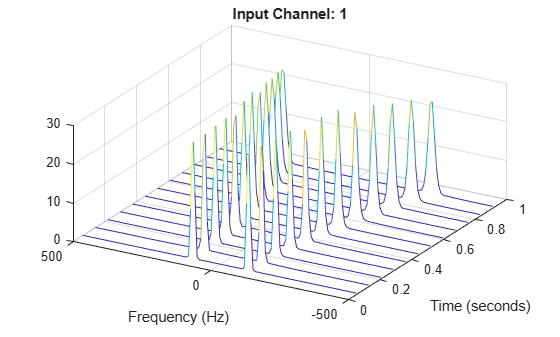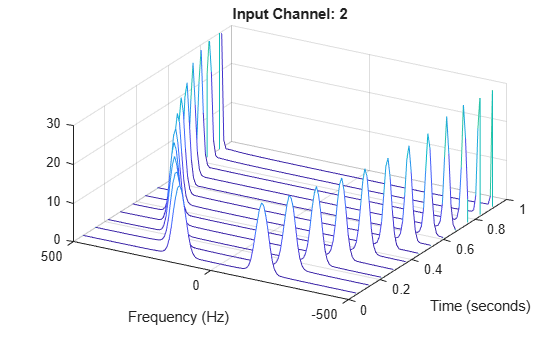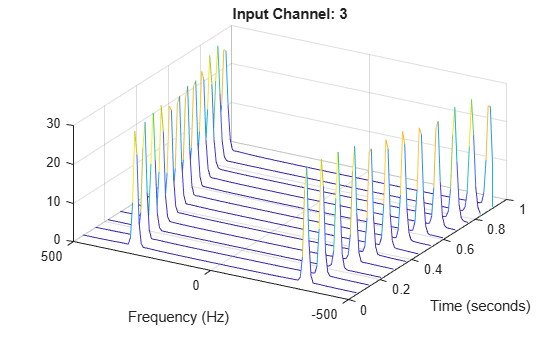# stft

Short-time Fourier transform

## Syntax

``s = stft(x)``
``s = stft(x,fs)``
``s = stft(x,ts)``
``s = stft(___,Name,Value)``
``[s,f] = stft(___)``
``[s,f,t] = stft(___)``
``stft(___)``

## Description

````s = stft(x)` returns the short-time Fourier transform (STFT) of `x`.```

example

````s = stft(x,fs)` returns the STFT of `x` using sample rate `fs`.```
````s = stft(x,ts)` returns the STFT of `x` using sample time `ts`.```

example

````s = stft(___,Name,Value)` specifies additional options using name-value pair arguments. Options include the FFT window and length. These arguments can be added to any of the previous input syntaxes.```
````[s,f] = stft(___)` returns the frequencies `f` at which the STFT is evaluated.```

example

````[s,f,t] = stft(___)` returns the times at which the STFT is evaluated.```
````stft(___)` with no output arguments plots the magnitude of the STFT in the current figure window. The STFT is plotted as two-sided and centered.```

## Examples

collapse all

Generate two seconds of a voltage controlled oscillator output, controlled by a sinusoid sampled at 10 kHz.

```fs = 10e3; t = 0:1/fs:2; x = vco(sin(2*pi*t),[0.1 0.4]*fs,fs);```

Compute and plot the STFT of the signal. Use a Kaiser window of length 256 and shape parameter $\beta =5$. Specify the length of overlap as 220 samples and DFT length as 512 points. Plot the STFT with default colormap and view.

`stft(x,fs,'Window',kaiser(256,5),'OverlapLength',220,'FFTLength',512);`Change the view to display the STFT as a waterfall plot. Set the colormap to `jet`.

```view(-45,65) colormap jet```Generate a quadratic chirp sampled at 1 kHz for 2 seconds. The instantaneous frequency is 100 Hz at $\mathit{t}=0$ and crosses 200 Hz at $\mathit{t}=1$ second.

```ts = 0:1/1e3:2; f0 = 100; f1 = 200; x = chirp(ts,f0,1,f1,'quadratic',[],'concave');```

Compute and display the STFT of the quadratic chirp with a duration of 1 ms.

```d = seconds(1e-3); win = hamming(100,'periodic'); stft(x,d,'Window',win,'OverlapLength',98,'FFTLength',128);```Generate a three-channel signal consisting of three different chirps sampled at 1 kHz for one second.

1. The first channel consists of a concave quadratic chirp with instantaneous frequency 100 Hz at t = 0 and crosses 300 Hz at t = 1 second. It has an initial phase equal to 45 degrees.

2. The second channel consists of a convex quadratic chirp with instantaneous frequency 200 Hz at t = 0 and crosses 600 Hz at t = 1 second.

3. The third channel consists of a logarithmic chirp with instantaneous frequency 300 Hz at t = 0 and crosses 500 Hz at t = 1 second.

Compute the STFT of the multichannel signal using a periodic Hamming window of length 128 and an overlap length of 50 samples.

```fs = 1e3; t = 0:1/fs:1-1/fs; x = [chirp(t,100,1,300,'quadratic',45,'concave'); chirp(t,200,1,600,'quadratic',[],'convex'); chirp(t,300,1,500,'logarithmic')]'; [S,F,T] = stft(x,fs,'Window',hamming(128,'periodic'),'OverlapLength',50);```

Visualize the STFT of each channel as a waterfall plot. Control the behavior of the axes using the helper function `helperGraphicsOpt`.

```waterfall(F,T,abs(S(:,:,1))') helperGraphicsOpt(1)``````waterfall(F,T,abs(S(:,:,2))') helperGraphicsOpt(2)``````waterfall(F,T,abs(S(:,:,3))') helperGraphicsOpt(3)```This helper function sets the appearance and behavior of the current axes.

```function helperGraphicsOpt(ChannelId) ax = gca; ax.XDir = 'reverse'; str = ['Input Channel: ',num2str(ChannelId)]; ax.Title.String = str; ax.YLabel.String = 'Frequency (Hz)'; ax.XLabel.String = 'Time (S)'; ax.View = [30 45]; end```

## Input Arguments

collapse all

Input signal, specified as a vector, a matrix, or a MATLAB® `timetable`.

### Note

If you invert `s` using `istft` and want the result to be the same length as `x`, the value of `(length(x)-noverlap)/(length(window)-noverlap)` must be an integer.

• If the input has multiple channels, specify `x` as a matrix where each column corresponds to a channel.

• For timetable input, `x` must contain uniformly increasing finite row times. If a timetable has missing or duplicate time points, you can fix it using the tips in Clean Timetable with Missing, Duplicate, or Nonuniform Times (MATLAB).

• For multichannel timetable input, specify `x` as a timetable with a single variable containing a matrix or a timetable with multiple variables each containing a column vector. All variables must have the same precision.

Each channel of `x` must have a length greater than the window length.

Example: `chirp(0:1/4e3:2,250,1,500,'quadratic')` specifies a single-channel chirp.

Example: `timetable(rand(5,2),'SampleRate',1)` specifies a two-channel random variable sampled at 1 Hz for 4 seconds.

Example: `timetable(rand(5,1),rand(5,1),'SampleRate',1)` specifies a two-channel random variable sampled at 1 Hz for 4 seconds.

Data Types: `double` | `single`
Complex Number Support: Yes

Sample rate, specified as a positive scalar. This argument applies only when `x` is a vector or a matrix.

Data Types: `double` | `single`

Sample time, specified as a `duration` scalar. This argument applies only when `x` is a vector or a matrix

Example: `seconds(1)` is a `duration` scalar representing a 1-second time difference between consecutive signal samples.

Data Types: `duration`

### Name-Value Pair Arguments

Specify optional comma-separated pairs of `Name,Value` arguments. `Name` is the argument name and `Value` is the corresponding value. `Name` must appear inside quotes. You can specify several name and value pair arguments in any order as `Name1,Value1,...,NameN,ValueN`.

Example: `stft('Window',win,'OverlapLength',50,'FFTLength',128)` windows the data using the window `win`, with 50 samples overlap between adjoining segments and 128 point FFT.

Spectral window, specified as the comma-separated pair consisting of `'Window'` and a vector. If you do not specify the window or specify it as empty, the function uses a Hann window of length 128. The length of `Window` must be greater than or equal to 2.

For a list of available windows, see Windows.

Example: `hann(N+1)` and `(1-cos(2*pi*(0:N)'/N))/2` both specify a Hann window of length `N` + 1.

Data Types: `double` | `single`

Number of overlapped samples, specified as the comma-separated pair consisting of `OverlapLength` and a positive integer smaller than the length of `window`. If you omit `'OverlapLength'` or specify it as empty, it is set to the largest integer less than 75% of the window length, which turns out to be 96 samples for the default Hann window.

Data Types: `double` | `single`

Number of DFT points, specified as the comma-separated pair consisting of `FFTLength` and a positive integer. The value must be greater than or equal to the window length. If the length of the input signal is less than the DFT length, the data is padded with zeros.

Data Types: `double` | `single`

Frequency range, specified as the comma-separated pair consisting of `Centered` and `true` or `false`. If this option is set to `true`, then the spectrum is centered and is computed over the interval π to π. Otherwise, the spectrum is computed over the interval 0 to 2π.

Output time dimension, specified as the comma-separated pair consisting of `OutputTimeDimension` and `acrosscolumns` or `downrows`. Set this value to `downrows`, if you want the time dimension of `s` down the rows and the frequency dimension along the columns. Set this value to `acrosscolumns`, if you want the time dimension of `s` across the columns and frequency dimension along the rows. This input is ignored if the function is called without output arguments.

## Output Arguments

collapse all

Short-time Fourier transform, returned as a matrix or a 3-D array. Time increases across the columns of `s` and frequency increases down the rows. The third dimension, if present, corresponds to the input channels.

• If the signal `x` has Nx time samples, then `s` has k columns, where k = ⌊(NxL)/(ML)⌋, M is the length of `'Window'`, L is the `'OverlapLength'`, and the ⌊ ⌋ symbols denote the floor function.

• The number of rows in `s` is equal to the value specified in `'FFTLength'`.

Data Types: `double` | `single`

Frequencies at which the STFT is evaluated, returned as a vector.

Data Types: `double` | `single`

Time instants, returned as a vector. `t` contains the time values corresponding to the centers of the data segments used to compute short-time power spectrum estimates.

• If a sample rate `fs` is provided, then the vector contains time values in seconds.

• If a sample time `ts` is provided, then the vector is a duration array with the same time format as the input.

• If no time information is provided, then the vector contains sample numbers.

Data Types: `double` | `single`

collapse all

### Short-time Fourier Transform

The short-time Fourier transform (STFT) is used to analyze how the frequency content of a nonstationary signal changes over time.

The STFT of a signal is calculated by sliding an analysis window of length $M$ over the signal and calculating the discrete Fourier transform of the windowed data. The window hops over the original signal at intervals of $R$ samples. Most window functions taper off at the edges to avoid spectral ringing. If a nonzero overlap length $L$ is specified, overlap-adding the windowed segments compensates for the signal attenuation at the window edges. The DFT of each windowed segment is added to a matrix that contains the magnitude and phase for each point in time and frequency. The number of rows in the STFT matrix equals the number of DFT points, and the number of columns is given by

`$k=⌊\frac{{N}_{x}-L}{M-L}⌋,$`

where ${N}_{x}$ is the length of the original signal $x\left(n\right)$ and the ⌊⌋ symbols denote the floor function.

The STFT matrix is given by $X\left(f\right)=\left[\begin{array}{ccccc}{X}_{1}\left(f\right)& {X}_{2}\left(f\right)& {X}_{3}\left(f\right)& \cdots & {X}_{k}\left(f\right)\end{array}\right]$ such that the $m$th element of this matrix is

`${X}_{m}\left(f\right)=\sum _{n=-\infty }^{\infty }x\left(n\right)g\left(n-mR\right){e}^{-j2\pi fn},$`

where

• $g\left(n\right)$ — Window function of length $M$.

• ${X}_{m}\left(f\right)$ — DFT of windowed data centered about time $mR$.

• $R$ — Hop size between successive DFTs. The hop size is the difference between the window length $M$and the overlap length $L$.

The magnitude squared of the STFT yields the `spectrogram` representation of the power spectral density of the function.### Perfect Reconstruction

In general, computing the STFT of an input signal and inverting it does not result in perfect reconstruction. If you want the output of ISTFT to match the original input signal as closely as possible, the signal and the window must satisfy the following conditions:

• Input size — If you invert the output of `stft` using `istft` and want the result to be the same length as the input signal `x`, the value of must be an integer.

• COLA compliance — Use COLA-compliant windows, assuming that you have not modified the short-time Fourier transform of the signal.

• Padding — If the length of the input signal is such that the value of k is not an integer, zero-pad the signal before computing the short-time Fourier transform. Remove the extra zeros after inverting the signal.

 Mitra, Sanjit K. Digital Signal Processing: A Computer-Based Approach. 2nd Ed. New York: McGraw-Hill, 2001.

 Smith, J. O. Spectral Audio Signal Processing. https://ccrma.stanford.edu/~jos/sasp/, online book, 2011 edition, accessed Nov 2018.

 Sharpe, Bruce. Invertibility of Overlap-Add Processing. https://gauss256.github.io/blog/cola.html, accessed July 2019.